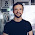### Java puzzle (Sum of even Fibonacci numbers)

Write a function which calculates the sum of even Fibonacci numbers.
```public static void main(String... args) {
for(int i=1;i<22;i++)
System.out.print(sumEvenFibonacci(i) + " ");
}

public static long sumEvenFibonacci(int n) {
return sum;
}
```
prints
```0 0 2 2 2 10 10 10 44 44 44 188 188 188 798 798 798 3382 3382 3382 14328
```
For bonus points, optimise the sumEvenFibonacci method so it contains only one condition and doesn't test for even or odd.

[Click for sample solution]

1.This should works
public static long sumEvenFibonacci(int n) {
long sum = 0;
long f1 = 0;
long f2 = 1;
for (int i = 0; i < n / 3; i++) {
f1 += 2 * f2;
f2 = 2 * f1 - f2;
sum += f1;
}
return sum;
}

2.@Eugen, That's a good solution. Can you do it without division or multiplication? ;) (Only the division wouldn't be optimised by the JIT)

3.A more traditional approach with some unsafe counter handling (to reduce the code size):
long sum = 0;
int f1 = 1;
int f2 = 0;
int counter = 1;
for (int i = 0; i < n; i++) {
if (counter % 3 == 0)
sum += f1;
f1 += f2;
f2 = f1 - f2;
counter++;
}
return sum;

But I really-really like Eugen's solution :)

4.Here's the safest solution:
if (counter % 3 == 0) {
counter = 0;
sum += f1;
}

5.if (counter == 3)

An absolute divisionless condition. Okok. I'm stopping now :)

6.Similar problem can be found at Project Euler. Solve to unlock "official" solution and discussion.

7.@Lexandro, It uses two conditions, do you need a "counter" if you have "i" ;)

8.This is the solution I came up with.

public static long sumEvenFibonacci(int n) {
long a = 1, b = 1, sum = 0;
for (int i = n; i > 2; i -= 3) {
long c = a + b;
sum += c;
a = b + c;
b = a + c;
}
return sum;
}

9.I don't know why, but when I use this for loop:

for (int i = 2; i < n; i += 3);

the code is 4% faster.

10.Finally I did a last test with a jre 7 and the same class files; in this case the fastest loop is:
for (int i = n; i > 2; i -= 3);

The JIT compilation result change from one VM to an other.

11.This comment has been removed by a blog administrator.

12.This comment has been removed by the author.